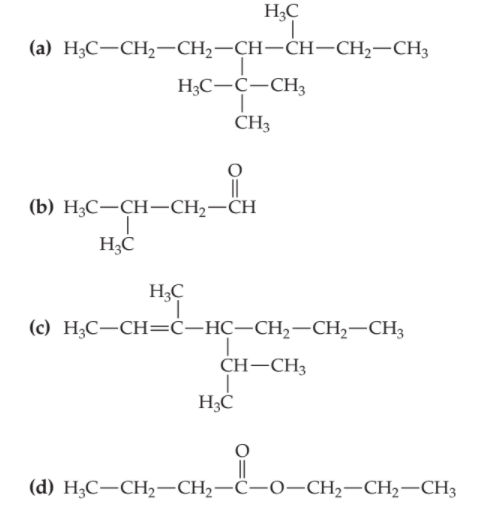×
Get Full Access to Introductory Chemistry - 5 Edition - Chapter 18 - Problem 105p
Get Full Access to Introductory Chemistry - 5 Edition - Chapter 18 - Problem 105p

×

# Name each compound.(a) (b) (c) (d)ISBN: 9780321910295 34

## Solution for problem 105P Chapter 18

Introductory Chemistry | 5th Edition

• Textbook Solutions
• 2901 Step-by-step solutions solved by professors and subject experts
• Get 24/7 help from StudySoup virtual teaching assistantsIntroductory Chemistry | 5th Edition

4 5 1 291 Reviews
18
5
Problem 105P

Name each compound.Step-by-Step Solution:
Step 1 of 3

Solution 105P:

Here, we need to name of each compound based on IUPAC (International Union of Pure and Applied Chemistry) nomenclature.

(a)The name of the compound is 3-methyl-4-tert- butylheptane.

(b)The name of the compound is 3-methylbutanal.

(c)The name of the compound is 3-methyl-4-isopropyl-2-heptene.

(d)The name of the compound is Propyl butanoate.

Step 2 of 3

Step 3 of 3

## Discover and learn what students are asking

Calculus: Early Transcendental Functions : Preparation for Calculus
?In Exercises 1–4, find any intercepts. $$y=(x-3) \sqrt{x+4}$$

Calculus: Early Transcendental Functions : Evaluating Limits Analytically
?Finding a Limit In Exercises 5-36, find the limit. $$\lim _{x \rightarrow 2} x^{3}$$

Calculus: Early Transcendental Functions : Introduction to Functions of Several Variables
?In Exercises 7-18, find and simplify the function values. $$f(x, y)=3 x^{2}-2 y$$ (a) $$\frac{f(x+\Delta x, y)-f(x, y)}{\Delta x}$$

Unlock Textbook Solution